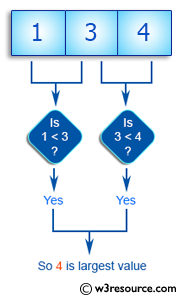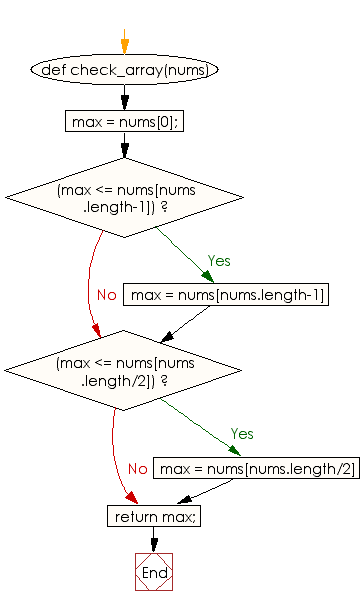﻿ Ruby Array exercises: Find the largest value from a given array of integers of odd length - w3resource# Ruby Array Exercises: Find the largest value from a given array of integers of odd length

## Ruby Array: Exercise-26 with Solution

Write a Ruby program to find the largest value from a given array of integers of odd length. The array length will be a least 1.Ruby Code:

``````def check_array(nums)
max = nums;
if(max <= nums[nums.length-1])
max = nums[nums.length-1]
end
if(max <= nums[nums.length/2])
max = nums[nums.length/2]
end
return max;
end

print check_array([1, 3, 4]),"\n"
print check_array([1, 2, 7]),"\n"
print check_array([1, 2])
``````

Output:

```4
7
2
```

Flowchart:Ruby Code Editor: